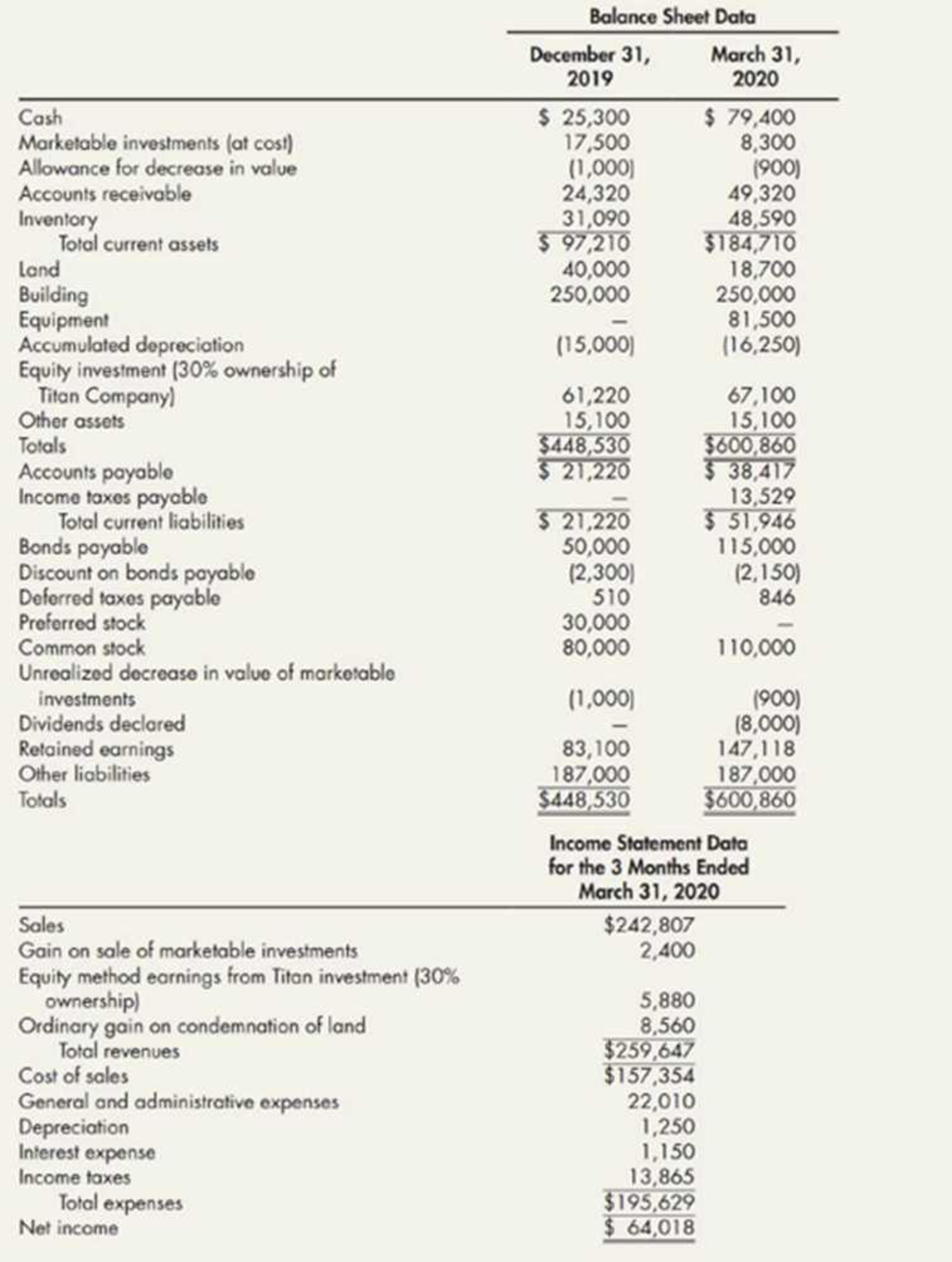Chapter 21, Problem 12P### Intermediate Accounting: Reporting...

3rd Edition
James M. Wahlen + 2 others
ISBN: 9781337788281

#### Solutions

Chapter
Section### Intermediate Accounting: Reporting...

3rd Edition
James M. Wahlen + 2 others
ISBN: 9781337788281
Textbook Problem
65 views

# Comprehensive Angel Company has prepared its financial statements for the year ended December 31, 2019, and for the 3 months ended March 31, 2020. You have been asked to prepare a statement of cash flows for the 3 months ended March 31, 2020. The company’s balance sheet data at December 31, 2019, and March 31, 2020, and its income statement data for the 3 months ended March 31, 2020, follow. You are satisfied as to the correctness of the amounts presented.Your discussion with the company’s controller and a review of the financial records have revealed the following information: a. On January 7, 2020, the company sold marketable securities for cash. These securities had cost $9,200, and had a fair value of$8,600 at December 31. 2019. The remaining marketable securities were adjusted to their $7,400 fair value on March 31, 2020, by adjustment of the related allowance account. The dividend and interest revenue on these marketable securities is not material. b. The company’s preferred stock was converted into common stock at a rate of one share of preferred for two shares of common. The preferred stock and common stock have par values of$2 and $1, respectively. c. On January 16, 2020, 3 acres of land were condemned. An award of$29,860 in cash was received on March 24, 2020. Purchase of additional land as a replacement is not contemplated by the company. d. On March 25, 2020, the company purchased equipment for cash. e. On March 26, 2020, bonds payable were issued by the company at par for cash. f. The equity investment representing a 30% ownership interest in Titan Company included an amount of $9,600 attributable to an increase in the recorded value of depreciable assets at December 31, 2019. This increase is being depreciated at a quarterly rate of$480. Required: 1. Prepare a spreadsheet to support the statement of cash flows for Angel for the 3 months ended March 31, 2020. 2. Prepare the statement of cash flows.

1.

To determine

Prepare a spreadsheet for the cash flow statement of A Company for the quarter ended March 31, 2020.

Explanation

Statement of cash flows: This statement reports all the cash transactions which are responsible for inflow and outflow of cash, and result of these transactions is reported as ending balance of cash at the end of reported period. Statement of cash flows includes the changes in cash balance due to operating, investing, and financing activities.

Worksheet: A worksheet is a spreadsheet used while preparing a financial statement. It is a type of form having multiple columns and it is used in the adjustment process. The use of a worksheet is optional for any organization. A worksheet can neither be considered as a journal nor a part of the general ledger.

Prepare a spreadsheet for the statement of cash flow of A Company.

Table (1)

Table (2)

Working notes:

a. Net income for the quarter ended is $64,018. b. c. Calculate the Depreciation expenses. d. Depreciation Expenses=(Beginning year's accumulated depreciation Ending year's accumulated depreciation )=$15,000$16,250=$1,250

e. Calculate the gain on sale of marketable investment.

(Proceeds from sale of Marketable Investments)=(Marketable Investments+Gain on sale of marketable investment)=($9,200+$2,400)=$11,600 f. Calculate the increase in accounts receivable. g. Increase in Accounts Receivable=(Beginning year's accounts receivableEnding year's accounts receivable)=$24,320$49,320=$25,000

h. Calculate the increase in inventory.

Increase in Inventory=(Beginning year's inventoryEnding year's inventory)=$31,090$48,590=$17,500 i. Calculate the increase in accounts payable. j. Depreciation Expenses=(Beginning year's accumulated depreciation Ending year's accumulated depreciation )=$15,000$16,250=$1,250

k. Income taxes payable is $13,529. h-1. Calculate the issuance of common stock for preferred stock. (Conversion of Preferred stock to Common stock)=(Beginning year's common stockEnding year's common stock)=$80,000$110,000=$30,000

h-2. Calculate the conversion of preferred stock to common stock is \$30,000.

i. Calculate the proceeds from condemnation of land

2.

To determine

Prepare the statement of cash flows using indirect method of A Company for the quarter ended March 31, 2019.

### Still sussing out bartleby?

Check out a sample textbook solution.

See a sample solution

#### The Solution to Your Study Problems

Bartleby provides explanations to thousands of textbook problems written by our experts, many with advanced degrees!

Get Started

#### What are the major functions of packaging?

Foundations of Business (MindTap Course List)

#### Why should policymakers think about incentives?

Principles of Microeconomics (MindTap Course List)

#### Indicate the effects of the transactions listed in the following table on total current assets, current ratio, ...

Fundamentals of Financial Management, Concise Edition (with Thomson ONE - Business School Edition, 1 term (6 months) Printed Access Card) (MindTap Course List)